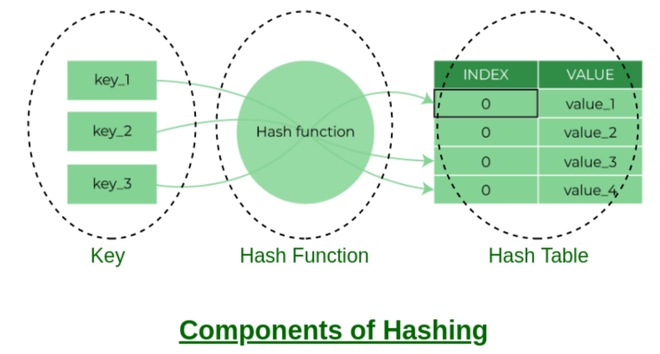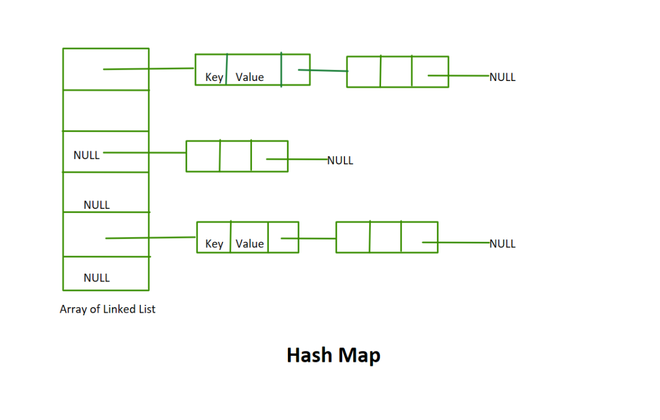GeeksforGeeks App
Open AppBrowser
Continue

# Implementation of Hash Table in C/C++ using Separate Chaining

### Introduction:

Hashing is a technique that maps a large set of data to a small set of data. It uses a hash function for doing this mapping. It is an irreversible process and we cannot find the original value of the key from its hashed value because we are trying to map a large set of data into a small set of data, which may cause collisions. It is not uncommon to encounter collisions when mapping a large dataset into a smaller one. Suppose, We have three buckets and each bucket can store 1L of water in it and we have 5L of water also. We have to put all the water in these three buckets and this kind of situation is known as a collision. URL shorteners are an example of hashing as it maps large size URL to small size

### Some Examples of Hash Functions:

• key % number of buckets
• ASCII value of character * PrimeNumberx. Where x = 1, 2, 3….n
• You can make your own hash function but it should be a good hash function that gives less number of collisions.Components of Hashing

### Bucket Index:

The value returned by the Hash function is the bucket index for a key in a separate chaining method. Each index in the array is called a bucket as it is a bucket of a linked list.

### Rehashing:

Rehashing is a concept that reduces collision when the elements are increased in the current hash table. It will make a new array of doubled size and copy the previous array elements to it and it is like the internal working of vector in C++. Obviously, the Hash function should be dynamic as it should reflect some changes when the capacity is increased. The hash function includes the capacity of the hash table in it, therefore, While copying key values from the previous array hash function gives different bucket indexes as it is dependent on the capacity (buckets) of the hash table. Generally,  When the value of the load factor is greater than 0.5 rehashings are done.

• Double the size of the array.
• Copy the elements of the previous array to the new array. We use the hash function while copying each node to a new array again therefore, It will reduce collision.
• Delete the previous array from the memory and point your hash map’s inside array pointer to this new array.
• Generally, Load Factor = number of elements in Hash Map / total number of buckets (capacity).

### Collision:

Collision is the situation when the bucket index is not empty. It means that a linked list head is present at that bucket index. We have two or more values that map to the same bucket index.

### Major Functions in our Program

• Insertion
• Search
• Hash Function
• Delete
• RehashingHash Map

Implementation without Rehashing:

## C

 `#include ``#include ``#include ` `// Linked List node``struct` `node {` `    ``// key is string``    ``char``* key;` `    ``// value is also string``    ``char``* value;``    ``struct` `node* next;``};` `// like constructor``void` `setNode(``struct` `node* node, ``char``* key, ``char``* value)``{``    ``node->key = key;``    ``node->value = value;``    ``node->next = NULL;``    ``return``;``};` `struct` `hashMap {` `    ``// Current number of elements in hashMap``    ``// and capacity of hashMap``    ``int` `numOfElements, capacity;` `    ``// hold base address array of linked list``    ``struct` `node** arr;``};` `// like constructor``void` `initializeHashMap(``struct` `hashMap* mp)``{` `    ``// Default capacity in this case``    ``mp->capacity = 100;``    ``mp->numOfElements = 0;` `    ``// array of size = 1``    ``mp->arr = (``struct` `node**)``malloc``(``sizeof``(``struct` `node*)``                                    ``* mp->capacity);``    ``return``;``}` `int` `hashFunction(``struct` `hashMap* mp, ``char``* key)``{``    ``int` `bucketIndex;``    ``int` `sum = 0, factor = 31;``    ``for` `(``int` `i = 0; i < ``strlen``(key); i++) {` `        ``// sum = sum + (ascii value of``        ``// char * (primeNumber ^ x))...``        ``// where x = 1, 2, 3....n``        ``sum = ((sum % mp->capacity)``               ``+ (((``int``)key[i]) * factor) % mp->capacity)``              ``% mp->capacity;` `        ``// factor = factor * prime``        ``// number....(prime``        ``// number) ^ x``        ``factor = ((factor % __INT16_MAX__)``                  ``* (31 % __INT16_MAX__))``                 ``% __INT16_MAX__;``    ``}` `    ``bucketIndex = sum;``    ``return` `bucketIndex;``}` `void` `insert(``struct` `hashMap* mp, ``char``* key, ``char``* value)``{` `    ``// Getting bucket index for the given``    ``// key - value pair``    ``int` `bucketIndex = hashFunction(mp, key);``    ``struct` `node* newNode = (``struct` `node*)``malloc``(` `        ``// Creating a new node``        ``sizeof``(``struct` `node));` `    ``// Setting value of node``    ``setNode(newNode, key, value);` `    ``// Bucket index is empty....no collision``    ``if` `(mp->arr[bucketIndex] == NULL) {``        ``mp->arr[bucketIndex] = newNode;``    ``}` `    ``// Collision``    ``else` `{` `        ``// Adding newNode at the head of``        ``// linked list which is present``        ``// at bucket index....insertion at``        ``// head in linked list``        ``newNode->next = mp->arr[bucketIndex];``        ``mp->arr[bucketIndex] = newNode;``    ``}``    ``return``;``}` `void` `delete` `(``struct` `hashMap* mp, ``char``* key)``{` `    ``// Getting bucket index for the``    ``// given key``    ``int` `bucketIndex = hashFunction(mp, key);` `    ``struct` `node* prevNode = NULL;` `    ``// Points to the head of``    ``// linked list present at``    ``// bucket index``    ``struct` `node* currNode = mp->arr[bucketIndex];` `    ``while` `(currNode != NULL) {` `        ``// Key is matched at delete this``        ``// node from linked list``        ``if` `(``strcmp``(key, currNode->key) == 0) {` `            ``// Head node``            ``// deletion``            ``if` `(currNode == mp->arr[bucketIndex]) {``                ``mp->arr[bucketIndex] = currNode->next;``            ``}` `            ``// Last node or middle node``            ``else` `{``                ``prevNode->next = currNode->next;``            ``}``            ``free``(currNode);``            ``break``;``        ``}``        ``prevNode = currNode;``        ``currNode = currNode->next;``    ``}``    ``return``;``}` `char``* search(``struct` `hashMap* mp, ``char``* key)``{` `    ``// Getting the bucket index``    ``// for the given key``    ``int` `bucketIndex = hashFunction(mp, key);` `    ``// Head of the linked list``    ``// present at bucket index``    ``struct` `node* bucketHead = mp->arr[bucketIndex];``    ``while` `(bucketHead != NULL) {` `        ``// Key is found in the hashMap``        ``if` `(bucketHead->key == key) {``            ``return` `bucketHead->value;``        ``}``        ``bucketHead = bucketHead->next;``    ``}` `    ``// If no key found in the hashMap``    ``// equal to the given key``    ``char``* errorMssg = (``char``*)``malloc``(``sizeof``(``char``) * 25);``    ``errorMssg = ``"Oops! No data found.\n"``;``    ``return` `errorMssg;``}` `// Drivers code``int` `main()``{` `    ``// Initialize the value of mp``    ``struct` `hashMap* mp``        ``= (``struct` `hashMap*)``malloc``(``sizeof``(``struct` `hashMap));``    ``initializeHashMap(mp);` `    ``insert(mp, ``"Yogaholic"``, ``"Anjali"``);``    ``insert(mp, ``"pluto14"``, ``"Vartika"``);``    ``insert(mp, ``"elite_Programmer"``, ``"Manish"``);``    ``insert(mp, ``"GFG"``, ``"GeeksforGeeks"``);``    ``insert(mp, ``"decentBoy"``, ``"Mayank"``);` `    ``printf``(``"%s\n"``, search(mp, ``"elite_Programmer"``));``    ``printf``(``"%s\n"``, search(mp, ``"Yogaholic"``));``    ``printf``(``"%s\n"``, search(mp, ``"pluto14"``));``    ``printf``(``"%s\n"``, search(mp, ``"decentBoy"``));``    ``printf``(``"%s\n"``, search(mp, ``"GFG"``));` `    ``// Key is not inserted``    ``printf``(``"%s\n"``, search(mp, ``"randomKey"``));` `    ``printf``(``"\nAfter deletion : \n"``);` `    ``// Deletion of key``    ``delete` `(mp, ``"decentBoy"``);``    ``printf``(``"%s\n"``, search(mp, ``"decentBoy"``));` `    ``return` `0;``}`

## C++

 `#include ``#include ` `// Linked List node``struct` `node {``    ``// key is string``    ``char``* key;``    ``// value is also string``    ``char``* value;``    ``struct` `node* next;``};` `// like constructor``void` `setNode(``struct` `node* node, ``char``* key, ``char``* value) {``    ``node->key = key;``    ``node->value = value;``    ``node->next = NULL;``    ``return``;``}` `struct` `hashMap {``    ``// Current number of elements in hashMap``    ``// and capacity of hashMap``    ``int` `numOfElements, capacity;``    ``// hold base address array of linked list``    ``struct` `node** arr;``};` `// like constructor``void` `initializeHashMap(``struct` `hashMap* mp) {``    ``// Default capacity in this case``    ``mp->capacity = 100;``    ``mp->numOfElements = 0;``    ``// array of size = 1``    ``mp->arr = (``struct` `node**)``malloc``(``sizeof``(``struct` `node*) * mp->capacity);``    ``return``;``}` `int` `hashFunction(``struct` `hashMap* mp, ``char``* key) {``    ``int` `bucketIndex;``    ``int` `sum = 0, factor = 31;``    ``for` `(``int` `i = 0; i < ``strlen``(key); i++) {``        ``// sum = sum + (ascii value of``        ``// char * (primeNumber ^ x))...``        ``// where x = 1, 2, 3....n``        ``sum = ((sum % mp->capacity) + (((``int``)key[i]) * factor) % mp->capacity) % mp->capacity;``        ``// factor = factor * prime``        ``// number....(prime``        ``// number) ^ x``        ``factor = ((factor % __INT16_MAX__) * (31 % __INT16_MAX__)) % __INT16_MAX__;``    ``}``    ``bucketIndex = sum;``    ``return` `bucketIndex;``}` `void` `insert(``struct` `hashMap* mp, ``char``* key, ``char``* value) {``    ``// Getting bucket index for the given``    ``// key - value pair``    ``int` `bucketIndex = hashFunction(mp, key);``    ``struct` `node* newNode = (``struct` `node*)``malloc``(``        ``// Creating a new node``        ``sizeof``(``struct` `node));``    ``// Setting value of node``    ``setNode(newNode, key, value);``    ``// Bucket index is empty....no collision``    ``if` `(mp->arr[bucketIndex] == NULL) {``        ``mp->arr[bucketIndex] = newNode;``    ``}``    ``// Collision``    ``else` `{``        ``// Adding newNode at the head of``        ``// linked list which is present``        ``// at bucket index....insertion at``        ``// head in linked list``        ``newNode->next = mp->arr[bucketIndex];``        ``mp->arr[bucketIndex] = newNode;``    ``}``    ``return``;``}` `void` `deleteKey(``struct` `hashMap* mp, ``char``* key) {``    ``// Getting bucket index for the``    ``// given key``    ``int` `bucketIndex = hashFunction(mp, key);``    ``struct` `node* prevNode = NULL;``    ``// Points to the head of``    ``// linked list present at``    ``// bucket index``    ``struct` `node* currNode = mp->arr[bucketIndex];``    ``while` `(currNode != NULL) {``        ``// Key is matched at delete this``        ``// node from linked list``        ``if` `(``strcmp``(key, currNode->key) == 0) {``            ``// Head node``            ``// deletion``            ``if` `(currNode == mp->arr[bucketIndex]) {``                ``mp->arr[bucketIndex] = currNode->next;``            ``}``            ``// Last node or middle node``            ``else` `{``                ``prevNode->next = currNode->next;``}``free``(currNode);``break``;``}` `prevNode = currNode;``        ``currNode = currNode->next;``    ``}``return``;``}` `char``* search(``struct` `hashMap* mp, ``char``* key) {``// Getting the bucket index for the given key``int` `bucketIndex = hashFunction(mp, key);``// Head of the linked list present at bucket index``struct` `node* bucketHead = mp->arr[bucketIndex];` `while` `(bucketHead != NULL) {``    ` `    ``// Key is found in the hashMap``    ``if` `(``strcmp``(bucketHead->key, key) == 0) {``        ``return` `bucketHead->value;``    ``}``    ` `    ``bucketHead = bucketHead->next;``}` `// If no key found in the hashMap equal to the given key``char``* errorMssg = (``char``*)``malloc``(``sizeof``(``char``) * 25);``strcpy``(errorMssg, ``"Oops! No data found.\n"``);``return` `errorMssg;``}`  `// Drivers code``int` `main()``{``// Initialize the value of mp``struct` `hashMap* mp = (``struct` `hashMap*)``malloc``(``sizeof``(``struct` `hashMap));``initializeHashMap(mp);``insert(mp, ``"Yogaholic"``, ``"Anjali"``);``insert(mp, ``"pluto14"``, ``"Vartika"``);``insert(mp, ``"elite_Programmer"``, ``"Manish"``);``insert(mp, ``"GFG"``, ``"GeeksforGeeks"``);``insert(mp, ``"decentBoy"``, ``"Mayank"``);` `printf``(``"%s\n"``, search(mp, ``"elite_Programmer"``));``printf``(``"%s\n"``, search(mp, ``"Yogaholic"``));``printf``(``"%s\n"``, search(mp, ``"pluto14"``));``printf``(``"%s\n"``, search(mp, ``"decentBoy"``));``printf``(``"%s\n"``, search(mp, ``"GFG"``));` `// Key is not inserted``printf``(``"%s\n"``, search(mp, ``"randomKey"``));` `printf``(``"\nAfter deletion : \n"``);` `// Deletion of key``deleteKey(mp, ``"decentBoy"``);` `// Searching the deleted key``printf``(``"%s\n"``, search(mp, ``"decentBoy"``));``return` `0;``}`

Output

```Manish
Anjali
Vartika
Mayank
GeeksforGeeks
Oops! No data found.

After deletion :
Oops! No data found.```

#### Explanation:

• insertion: Inserts the key-value pair at the head of a linked list which is present at the given bucket index.
• hashFunction: Gives the bucket index for the given key. Our hash function = ASCII value of character * primeNumberx. The prime number in our case is 31 and the value of x is increasing from 1 to n for consecutive characters in a key.
• deletion: Deletes key-value pair from the hash table for the given key. It deletes the node from the linked list which holds the key-value pair.
• Search: Search for the value of the given key.
• This implementation does not use the rehashing concept. It is a fixed-sized array of linked lists.
• Key and value both are strings in the given example.

### Time Complexity and Space Complexity:

The time complexity of hash table insertion and deletion operations is O(1) on average. There is some mathematical calculation that proves it.

• Time Complexity of Insertion: In the average case it is constant. In the worst case, it is linear.
• Time Complexity of Search: In the average case it is constant. In the worst case, it is linear.
• Time Complexity of Deletion: In average cases it is constant. In the worst case, it is linear.
• Space Complexity: O(n) as it has n number of elements.

Related Articles:

My Personal Notes arrow_drop_up